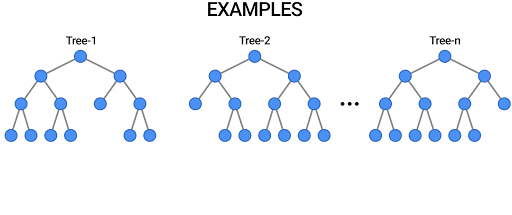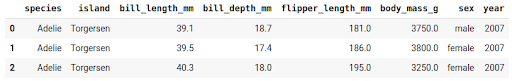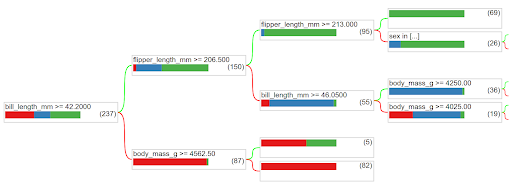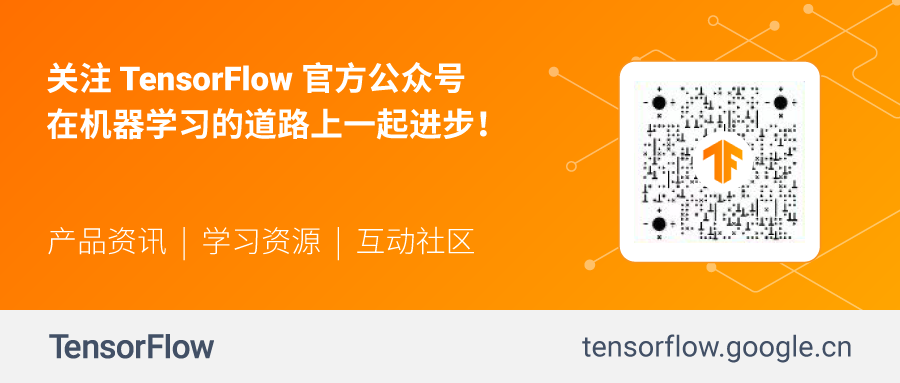﻿• 价格透明
• 信息保密
• 进度掌控
• 售后无忧# TensorFlow 决策森林来啦！# 决策森林简介

TF-DF 为 TensorFlow 用户提供了这类模型以及整套量身定制的工具：

• 初学者会发现开发和解释决策森林模型更为轻松。无需显式列出或预处理输入特征（因为决策森林可以自然处理数字属性和分类属性），无需指定架构（例如，相较于神经网络，无需通过尝试不同的层组合来指定架构），也无需担心模型发散。训练好模型后，您就可以直接对其进行绘制，或使用易于解释的统计数据对其分析。

• 高级用户将从推理速度非常快的模型中受益（在多数情况下每个示例的推理时间为亚微秒级）。同时，该库为模型实验和研究提供了大量的可组合性。特别是，用户可以很轻松地将神经网络和决策森林结合起来。

• 它提供一系列最先进的决策森林训练和应用算法，例如随机森林、梯度提升树、CART、(Lambda)MART、DART、极端随机树、Greedy Global Growth、Oblique Tree、单边采样、分类集学习、随机分类学习、包外评估、特征重要性以及结构特征重要性。

• 可连通丰富的 TensorFlow 生态系统，使您能够更轻松地将基于决策树的模型与各种 TensorFlow 工具、库以及 TFX 等平台集成。

• 如果你还没有接触过神经网络，则可以通过决策森林轻松入门 TensorFlow，并以此为起点，继续深入探索神经网络。

# 代码示例

## 训练模型``````#安装 TensorFlow 决策森林
!pip install tensorflow_decision_forests

#加载 TensorFlow 决策森林
import tensorflow_decision_forests as tfdf

#使用 Pandas 加载训练数据集
import pandas

#将 Pandas Dataframe 转换为 TensorFlow 数据集
train_ds = tfdf.keras.pd_dataframe_to_tf_dataset(train_df, label="species")

#训练模型
model = tfdf.keras.RandomForestModel()
model.fit(train_ds)``````

## 评估模型

``````#加载测试数据集

#将其转换为 TensorFlow 数据集
test_ds = tfdf.keras.pd_dataframe_to_tf_dataset(test_df, label="species")

#评估模型
model.compile(metrics=["accuracy"])
print(model.evaluate(test_ds))
# >> 0.979311
#注意：交叉验证更适合这样的小型数据集。
#另请参阅下方的“包外评估”。

#将模型导入 TensorFlow SavedModel
model.save("project/my_first_model")``````

## 解释模型

``tfdf.model_plotter.plot_model_in_colab(model, tree_idx=0)``• 每个特征使用了多少次？

• 模型训练的速度有多快（按决策树的数量和时间）？

• 树结构中的节点是如何分布的（例如，大多数分支的长度是多少？）

``````#打印关于模型的所有可用信息
model.summary()
>> Input Features (7):
>>   bill_depth_mm
>>   bill_length_mm
>>   body_mass_g
>>   ...
>> Variable Importance:
>>   1.    "bill_length_mm" 653.000000 ################
>>   ...
>> Out-of-bag evaluation: accuracy:0.964602 logloss:0.102378
>> Number of trees: 300
>> Total number of nodes: 4170
>>   ...

#以数组形式获取特征的重要性
model.make_inspector().variable_importances()["MEAN_DECREASE_IN_ACCURACY"]
>> [("flipper_length_mm", 0.149),
>>      ("bill_length_mm", 0.096),
>>      ("bill_depth_mm", 0.025),
>>      ("body_mass_g", 0.018),
>>      ("island", 0.012)]``````

``````#列出所有其他可用的学习算法
tfdf.keras.get_all_models()
>> [tensorflow_decision_forests.keras.RandomForestModel,
>>  tensorflow_decision_forests.keras.CartModel]

#显示梯度提升树模型的超参数
>> A GBT (Gradient Boosted [Decision] Tree) is a set of shallow decision trees trained sequentially. Each tree is trained to predict and then "correct" for the errors of the previously trained trees (more precisely each tree predicts the gradient of the loss relative to the model output)..
...
Attributes:
num_trees: num_trees: Maximum number of decision trees. The effective number of trained trees can be smaller if early stopping is enabled. Default: 300.
max_depth: Maximum depth of the tree. `max_depth=1` means that all trees will be roots. Negative values are ignored. Default: 6.
...

#使用指定的超参数创建另一个模型
num_trees=500,
growing_strategy="BEST_FIRST_GLOBAL",
max_depth=8,
split_axis="SPARSE_OBLIQUE",
)

#评估模型
model.compile(metrics=["accuracy"])
print(model.evaluate(test_ds))
# >> 0.986851``````

# 您的下一步

• 可以在初学者 Colab Notebook 中找到这篇文章的完整代码，也可以查看此处的中级 Notebook 和高级 Notebook。

• 观看 Google I/O 大会上的视频：Decision forests in TensorFlow | Session。

• 在 GitHub 上查看 TensorFlow 决策森林项目并 Star。

• 如果您已经在使用 TensorFlow 且有兴趣将决策森林添加到现有工作流程中，可阅读我们的迁移指南获取更多实用信息。

• 高级用户也可以关注 Yggdrasil Decision ForestsYggdrasil Decision Forest GitHub 项目，即由 C++ 引擎提供支持的 TensorFlow 决策森林，其中已经实现了该算法。

# 推荐阅读

### 使用 MoveNet 和 TensorFlow.js 的下一代姿态检测### 低价透明### 金牌服务### 信息保密